The K5 Learning Blog urges parents to be pro-active in helping their children reach their full academic potential.

# Math Worksheets for Kids in Kindergarten

In the first year of school, kindergarten students learn simple numbers and operations concepts, starting with numbers and counting and ending the year with simple addition and subtraction. To that end, we have created hundreds of simple math worksheets for your 5-year-olds to practice.

## Numbers and counting

The worksheets in this section are categorized by learning numbers, counting, odd/even numbers, ordinal numbers, more than/less than worksheets. All worksheets are colorful and focused on one concept at a time. Here’s one of our learn to print numbers worksheets: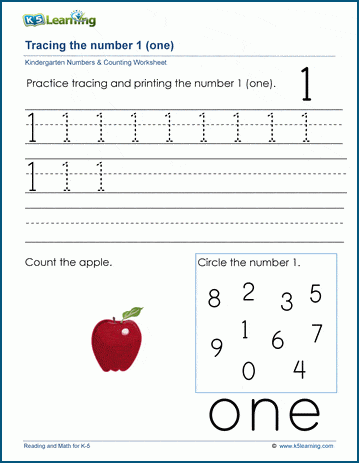## Patterns

We’ve created three sections of patterns worksheets: patterns of shapes, patterns of objects and patterns of letters.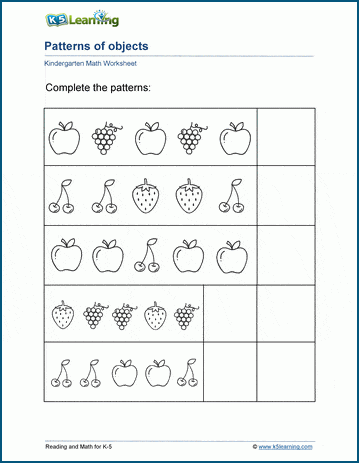## Measurement

Kindergarten students learn simple measurement concepts, such as measuring objects against a scale, and measuring and coloring given lengths.## Money

Students learn to recognize coins, their values and counting small groups of coins.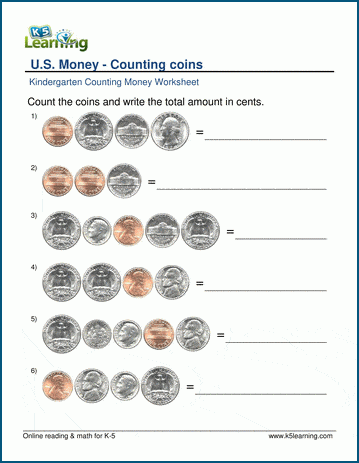## Graphing

We’ve created simple graphing worksheets that focus on reading bar charts, sorting objects and creating bar charts, and answering questions about given bar charts.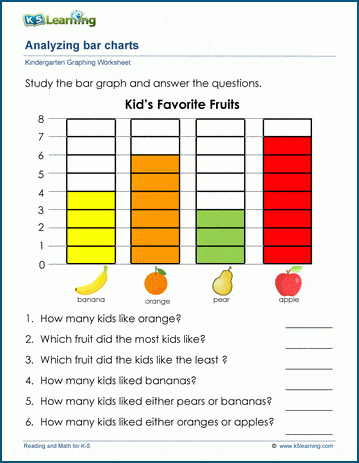As this is an important area for kindergarten, we have put effort into creating several categories of simple addition worksheets from simple counting objects to adding numbers up to the sum of 20.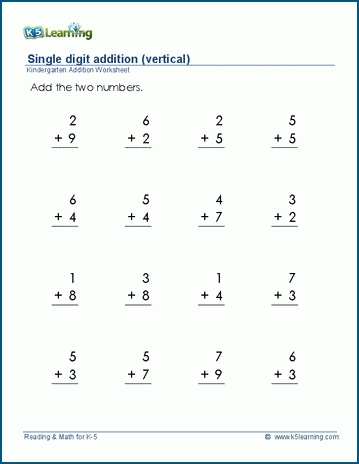## Subtraction

Similarly for subtraction, we have created several categories for kids to practice this important math skill.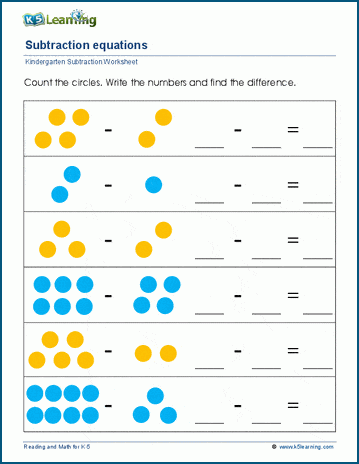## Math workbooks for kindergarten

For even further practice, we have also published a series of 5 math workbooks for kindergarten.

Measurement, time and money

We also sell a bundle of the five math workbooks at a discount.